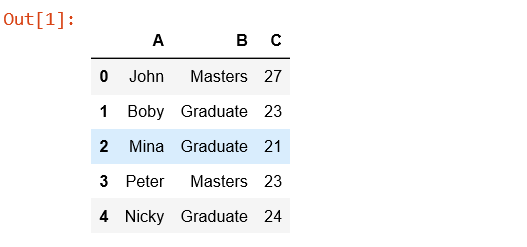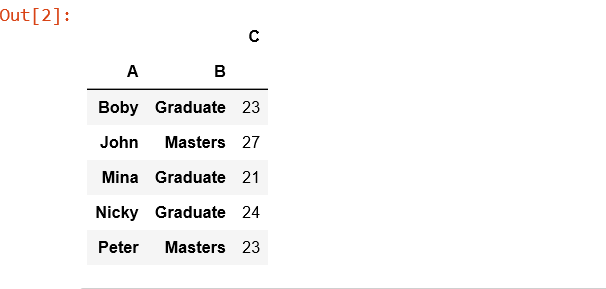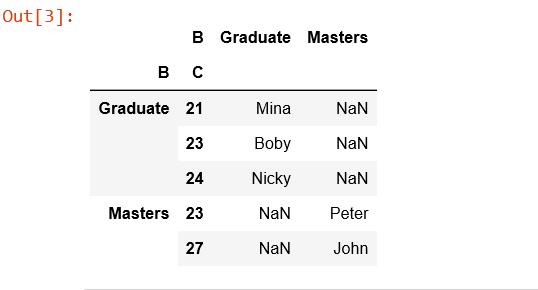Skip to content
Related Articles
Python | Pandas.pivot_table()
• Last Updated : 28 Sep, 2018

pandas.pivot_table(data, values=None, index=None, columns=None, aggfunc=’mean’, fill_value=None, margins=False, dropna=True, margins_name=’All’) create a spreadsheet-style pivot table as a DataFrame.

Levels in the pivot table will be stored in MultiIndex objects (hierarchical indexes) on the index and columns of the result DataFrame.

`Parameters:`

data : DataFrame
values : column to aggregate, optional
index: column, Grouper, array, or list of the previous
columns: column, Grouper, array, or list of the previous

aggfunc: function, list of functions, dict, default numpy.mean
-> If list of functions passed, the resulting pivot table will have hierarchical columns whose top level are the function names.
-> If dict is passed, the key is column to aggregate and value is function or list of functions

fill_value[scalar, default None] : Value to replace missing values with
margins[boolean, default False] : Add all row / columns (e.g. for subtotal / grand totals)
dropna[boolean, default True] : Do not include columns whose entries are all NaN
margins_name[string, default ‘All’] : Name of the row / column that will contain the totals when margins is True.

`Returns:` DataFrame

Code:

 `# Create a simple dataframe``  ` `# importing pandas as pd``import` `pandas as pd``import` `numpy as np``  ` `# creating a dataframe``df ``=` `pd.DataFrame({``'A'``: [``'John'``, ``'Boby'``, ``'Mina'``, ``'Peter'``, ``'Nicky'``],``      ``'B'``: [``'Masters'``, ``'Graduate'``, ``'Graduate'``, ``'Masters'``, ``'Graduate'``],``      ``'C'``: [``27``, ``23``, ``21``, ``23``, ``24``]})``  ` `df``# Simplest pivot table must have a dataframe``# and an index/list of index.``table ``=` `pd.pivot_table(df, index ``=``[``'A'``, ``'B'``])`` ` `table``# Creates a pivot table dataframe``table ``=` `pd.pivot_table(df, values ``=``'A'``, index ``=``[``'B'``, ``'C'``],``                         ``columns ``=``[``'B'``], aggfunc ``=` `np.``sum``)`` ` `table`Attention geek! Strengthen your foundations with the Python Programming Foundation Course and learn the basics.

To begin with, your interview preparations Enhance your Data Structures concepts with the Python DS Course. And to begin with your Machine Learning Journey, join the Machine Learning – Basic Level Course

My Personal Notes arrow_drop_up